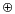Previous | ToC | Next Labs: Error Correction and Compression. Part 1. Math Alive

## Hamming Code

Repeating every bit three times does allow us to detect and correct errors, but it means that we need three times as much memory! That is why mathematicians work to develop other error correcting codes, or ways of encoding bit strings into slightly longer bit strings, which have enough redundancy so that errors can be corrected. A simple example is the 4-7 Hamming code which we discuss below.

This error correcting code replaces every string of 4 bits by a string of 7 bits (this still means that we need more memory than for the raw, uncoded data, but we need slightly less than twice the original space, versus three times in our tripling example above).

This is how it works:
given a 4 bit string b1 b2 b3 b4, we construct a 7 bit string: b1 b2 b3 b4 b5 b6 b7; the first 4 bits are just the old 4 bits again, and the new bits b5, b6 and b7 are computed by means of our old friend parity addition:

 b5 = b1b2b3 b6 = b1b3b4 b7 = b2b3b4

Every possible string of 7 bits that can be constructed in this way is called a codeword. Let's do a few examples. Find the codewords corresponding to these four bit combinations. After typing in your answer, press return to see if you are correct:

Practice
Hamming Encoding